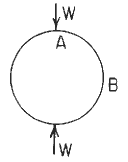Related Resources: calculators

### Circular Ring Stress and Deformations Formulae and Calculator

Per. Roarks Formulas for Stress and Strain Formulas for Circular Rings Section 9Circular Ring LoadingCircular Ring Dimensional PropertiesResultant moment, hoop load, and radial shear

Moment
M = MA - NA R ( 1 - u) + VA R z + LTM

Hoop Stress
N = NA u + Va z + LTN

V = - NA z + VA u + LTv

LTM LTN, and LTV are load terms for several types of load.

LTM = ( - W R z ) / 2

LTN = ( - W z ) / 2

LTv = ( - W u ) / 2

MA = ( W R k2 ) / π

NA = 0

VA = 0

Ri / t ≥ 10 means thin wall for pressure vessels (per. ASME Pressure Vessel Code)

Hoop Stress Deformation Factor α

α = e / R for thick rings
α = I / AR2 for thin rings
A = π [ R2 - Ri2 ]

Transverse (radial) shear deformation factor β

β = 2F (1 + v) e / R for thick rings
β = FEI / GAR2 for thin rings

k1 = 1 - α + β

k2 = 1 - α

Plastic section modulus
F = Z / I c
Z = Zx = Zy = 1.33R3
Ix = Iy = ( π / 4 ) ( R4 - Ri4)

Deformation in the Horizontal AxisDeformation in the Vertical AxisWhere (when used in equations and this calculator):

v and w = unit loads (force per unit of circumferential length);
G = Shear modulus of elasticity
F = Shape factor for the cross section (= Z / I c)
Z = Plastic section modulus
w and v = unit loads (force per unit of circumferential length);
ρ = unit weight of contained liquid (force per unit volume);
Mo = applied couple (force-length);
MA, MB, Mc, and M are internal moments at A;B;C, and x, respectively, positive as shown.
NA N, VA, and V are internal forces, positive as shown.
E = modulus of elasticity (force per unit area);
v = Poisson’s ratio;
A = cross-sectional area (length squared);
R = Outside radius to the centroid of the cross section (length);
Ri = Inside radius to the centroid of the cross section;
t = wall thickness
I = area moment of inertia of ring cross section about the principal axis perpendicular to the plane of the ring (length4). [Note that for a pipe or cylinder, a representative segment of unit axial length may be used by replacing EI by Et3 / 12 (1 - v2 )
e ≈ I / ( R A) positive distance measured radially inward from the centroidal axis of the cross section to the neutral axis of pure bending
θ, x, and Φ are angles (radians) and are limited to the range zero to π for all cases except 18 and 19
s = sin θ
c = cos θ
z = sin x,
u = cos x
n = sin Φ
m = cos Φ
α - Hoop stress deformation factor
ΔDV = Change in vertical diameter (in, mm),
ΔDH = Change in horizontal diameter (in, mm),
ΔL = Change in lower half of vertical diameterthe vertical motion realative to point C of a line connecting points B and D on the ring,
ΔLW = Vertical motion relative to point C of a horizontal line connecting the load points on the ring,
ΔLWH = Change in length of a horizontal line connecting the load points on the ring,
ψ = angular rotation (radians) of the load point in the plane of the ring and is positive in the direction of positive θ.

Reference:

Roarks Formulas for Stress and Strain, 7th Edition